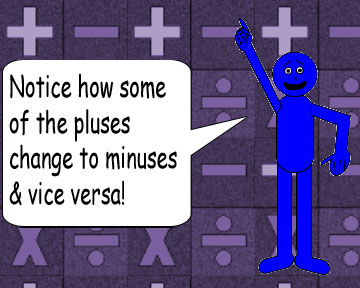# Cuckoo For Conjugates 2!

## Examples:

√(-i) = √(2)/2 - √(2)/2i & √(i) = √(2)/2 + √(2)/2i

√(-9i) = 3√(2)/2 - 3√(2)/2i & √(9i) = 3√(2)/2 + 3√(2)/2i

√(-5i) = √(5/2) - √(5/2)i & √(5i) = √(5/2) + √(5/2)i

√(-7i) = √(7/2) - √(7/2)i & √(7i) = √(7/2) + √(7/2)i

√(-1.5i) = √(3/2) - √(3/2)i & √(1.5i) = √(3/2) + √(3/2)i

√(-πi) = √(π/2) - √(π/2)i & √(πi) = √(π/2) + √(π/2)iIt doesn't matter what real number you plug in for the variable b, this trick will still work out! And remember: real × imaginary = imaginary!According to the examples above, the real part & the imaginary part of each complex number is likely to be a fraction with the number 2 as the denominator! But if you pick an even number, then...

√(-2i) = 1 - i & √(2i) = 1 + i

√(-8i) = 2 - 2i & √(8i) = 2 + 2i

√(-36i) = 3√(2) - 3√(2)i & √(36i) = 3√(2) + 3√(2)i

...The real part & the imaginary part won't be fractions, but they're still likely to be multiples of the square root of 2. Furthermore, both parts of the complex number will always equal each other.